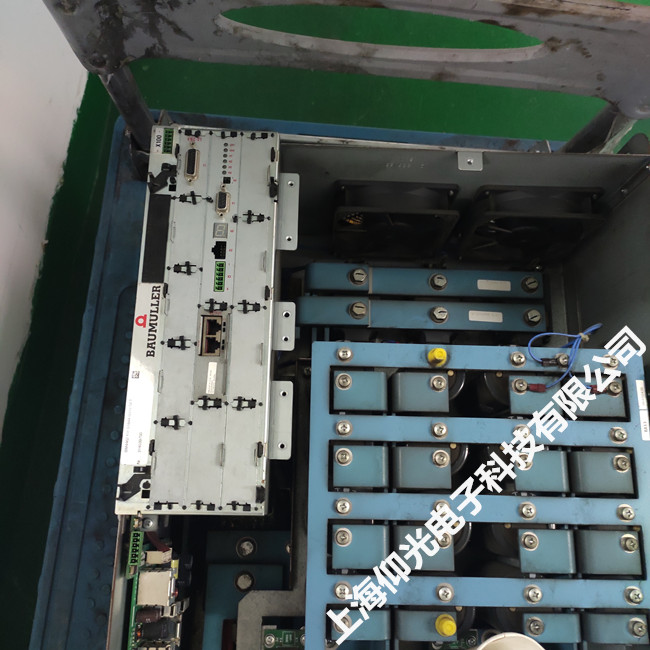Hi，欢迎访问上海仰光电子科技有限公司！

## 包米勒 bm4400驱动器故障报警P214P214

208=堵转
209=编码器1被参数化为电机控制的编码器，但评估未被激活。 如果错误定位在一个非活动数据记录中，则也会发生此错误
210=编码器2被参数化为电机控制的编码器，但评估未被激活。 如果错误定位在一个非活动数据记录中，则也会发生此错误
211=超速开环
212-223=保留

P215

224-234=保留
235=扭矩耦合：主控中的一般错误
236=扭矩耦合：从站的运行模式不是速度控制
237 =C配置错误反应返回无效
238=返回运动目的地没有被重新定位
239=应用程序错误（由P0302位1启用）

P216
CAN同步控制故障：
240-245=保留
246=无效的DIP开关设置
247-255=保留

P0233

6=数据溢出
7=位帧错误
8=命令状态无效
9=奇偶校验错误
10=校验和错误
11=未知的错误代码
12=数据编号错误
13=无效的论点
14=数据字段被写保护
15=访问代码无效
16=数据字段的大小不可更改错误
17=数据字段外的字地址错误
18=数据字段不存在
36=错误的数据校验和
37=没有反应
66=响应无效

P0234
P0235

1=超出规格的模拟信号。检查编码器电缆以及编码器是否已正确连接
2=内角偏移误差
3=数据字段分区表已损坏
4=模拟限值不可用
5=内部I2C总线无法运行
6=内部校验和错误
7=内部看门狗错误
8=计数器溢出
9=奇偶校验错误

10=校验和错误
11=未知的错误代码
12=数据编号错误
13=无效的ent参数
14=数据字段被写保护
15=访问代码无效
16=数据字段的大小不可更改
17=数据字段之外的字地址
18=数据字段不存在
19-27=保留
28=绝对监控模拟信号。检查编码器电缆以及编码器是否已正确连接
29=关键的传输电流
30=编码器温度至关重要
31=转速太高 - 没有形成可能的定位
32=位置单圈不可靠
33-35=多圈位置错误
36=无效的功率单元数据校验和
37=编码器无响应
38=编码器地址未知
39=读取绝对角度位置时出错
40=接收数据的校验和无效
41=编码器类型未知
42-63=保留
64=HIPERFACE编码器无响应
65=EnDat编码器无响应
66=对编码器命令无用的响应
67=编码器类型不适用
68-79=保留
80=CRC确定了一个错误
81=命令无效
82=响应电报出错
83=报警位已设置
84=内存被占用
85=数据校验和不正确
86=电机数据长度和/或编码器和控制器固件的数据版本不相同
87=没有EnDat接口
88=超过可评估的传输格式
89=超过可评估的测量步长
90=信号周期长度<测量步长错误）
91=EnDat 2.2：初始化主模块时出错
92=EnDat 2.2：测量信号传播时间的超时
93=EnDat 2.2：错误 - 关闭传播时间补偿
94=EnDat 2.2：编码器类型不支持EnDat2.2（引言集，电源，时钟频率）
95=EnDat 2.2：未设置RM位，未参考编码器绝对位置
96=错误照明
97=误差信号幅度
98=错误位置值
99=错误过电压
100=欠压错误
101=错误过流
102=电池错误
103-111=保留
112=多次请求期间检测到位置错误
113=附加信息触发的错误1
114=附加信息触发的错误2
115=附加信息触发的错误3
116=附加信息触发的错误4
117=附加信息触发的错误5
118=附加信息触发的错误6
119=附加信息触发的错误7

【 我们确保修好测试好给客户！！！！】